# Phase Diagrams AP CHEMISTRY Phase Diagrams Phase diagrams

• Slides: 30Phase Diagrams AP CHEMISTRY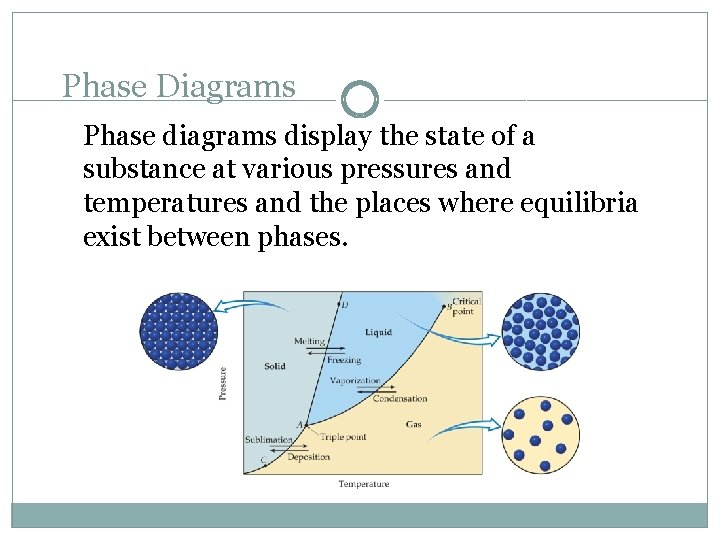Phase Diagrams Phase diagrams display the state of a substance at various pressures and temperatures and the places where equilibria exist between phases.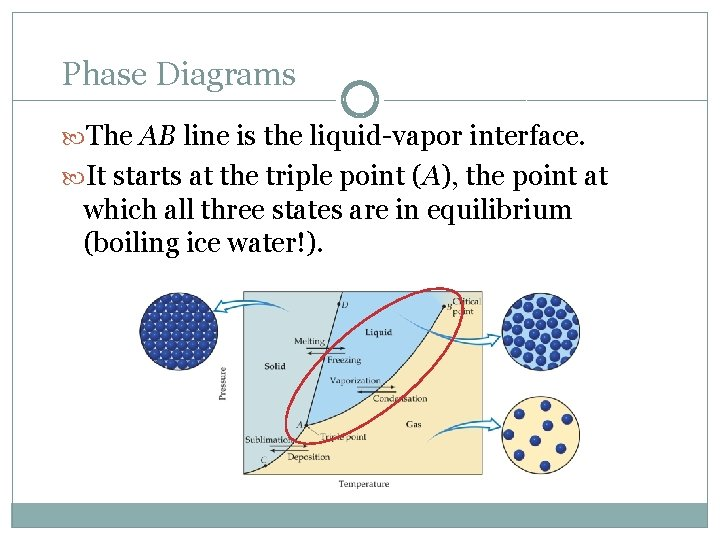Phase Diagrams The AB line is the liquid-vapor interface. It starts at the triple point (A), the point at which all three states are in equilibrium (boiling ice water!).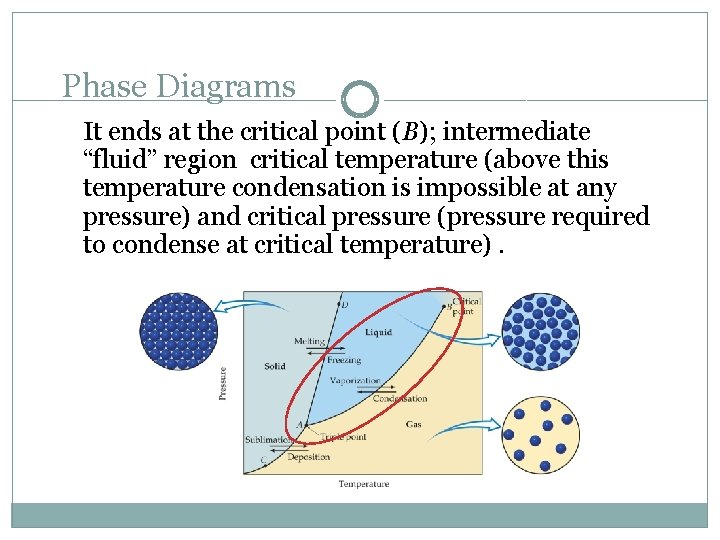Phase Diagrams It ends at the critical point (B); intermediate “fluid” region critical temperature (above this temperature condensation is impossible at any pressure) and critical pressure (pressure required to condense at critical temperature).Phase Diagrams Each point along this line is the boiling point of the substance at that pressure.Phase Diagrams The AD line is the interface between liquid and solid. The melting point at each pressure can be found along this line.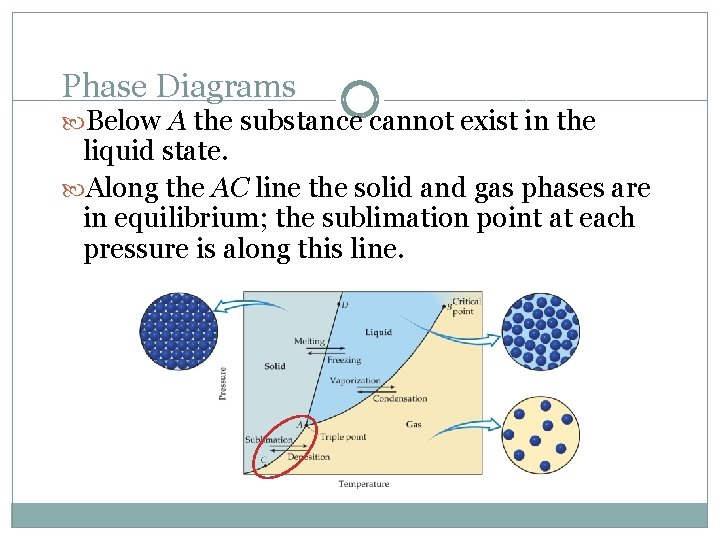Phase Diagrams Below A the substance cannot exist in the liquid state. Along the AC line the solid and gas phases are in equilibrium; the sublimation point at each pressure is along this line.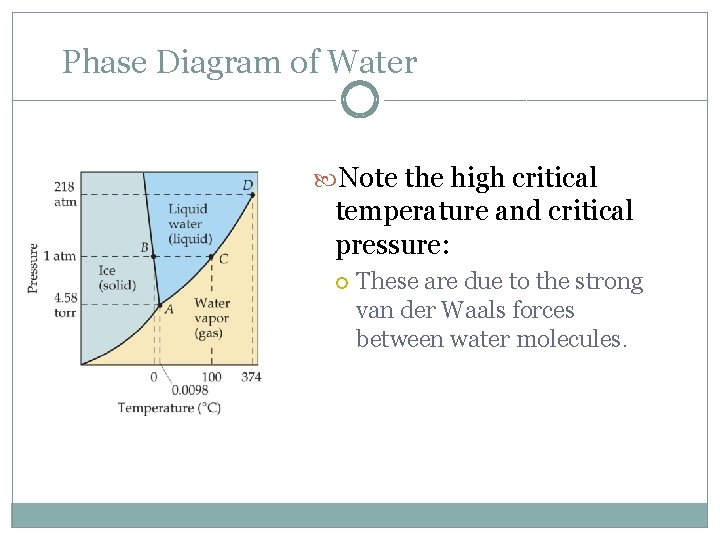Phase Diagram of Water Note the high critical temperature and critical pressure: These are due to the strong van der Waals forces between water molecules.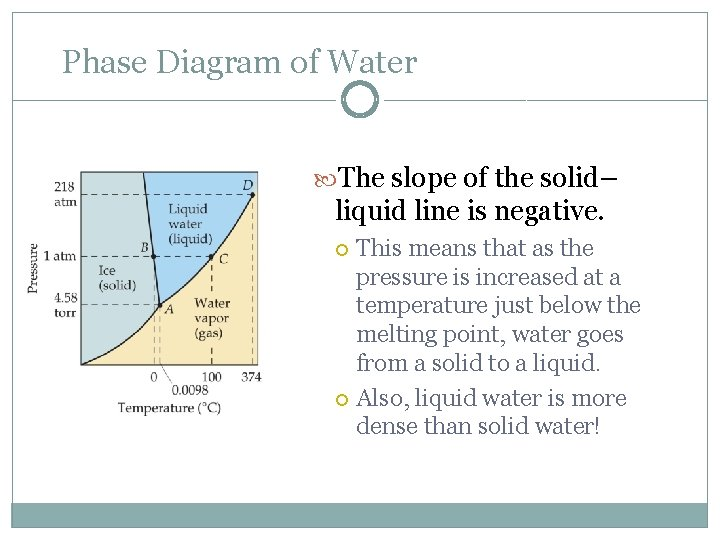Phase Diagram of Water The slope of the solid– liquid line is negative. This means that as the pressure is increased at a temperature just below the melting point, water goes from a solid to a liquid. Also, liquid water is more dense than solid water!Phase Diagram of Carbon Dioxide Carbon dioxide cannot exist in the liquid state at pressures below 5. 11 atm; CO 2 sublimes at normal pressures.Phase Diagram of Carbon Dioxide The low critical temperature and critical pressure for CO 2 make supercritical CO 2 a good solvent for extracting nonpolar substances (such as caffeine).Phase Diagram for Carbon: 1. Can we change pencil graphite into diamonds? 2. How many triple points?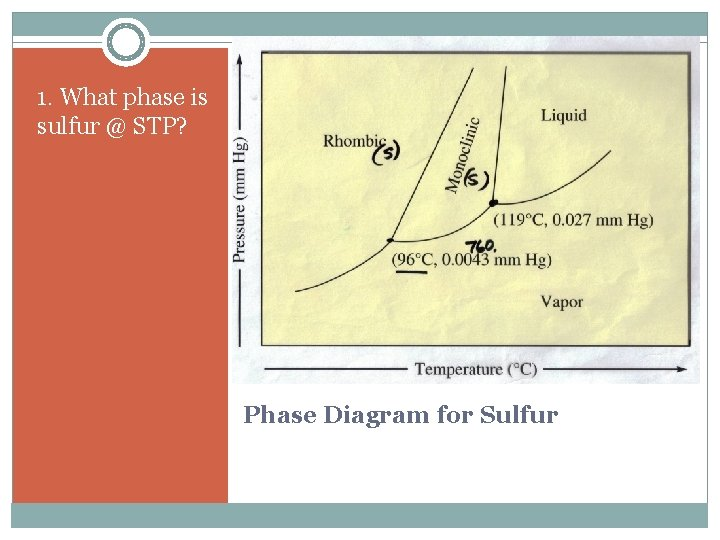1. What phase is sulfur @ STP? Phase Diagram for SulfurOf the following substances, predict which has the highest boiling point based on intermolecular forces. 1. Propane, C 3 H 8 2. Dimethyl ether, CH 3 OCH 3 3. Methyl chloride, CH 3 Cl 4. Acetaldehyde, CH 3 CHO 5. Acetonitrile, CH 3 CNCorrect Answer: 1. Propane, C 3 H 8 2. Dimethyl ether, CH 3 OCH 3 3. Methyl chloride, CH 3 Cl 4. Acetaldehyde, CH 3 CHO 5. Acetonitrile, CH 3 CN Each of these molecules has almost the same molecular weight; however, acetonitrile has the largest dipole moment (3. 9 D) and hence the largest dipole-dipole forces. Thus it has the highest boiling point.Of the following substances, predict which has the lowest boiling point based on London dispersion forces. 1. 2. 3. 4. 5. He Ne Ar Kr Xe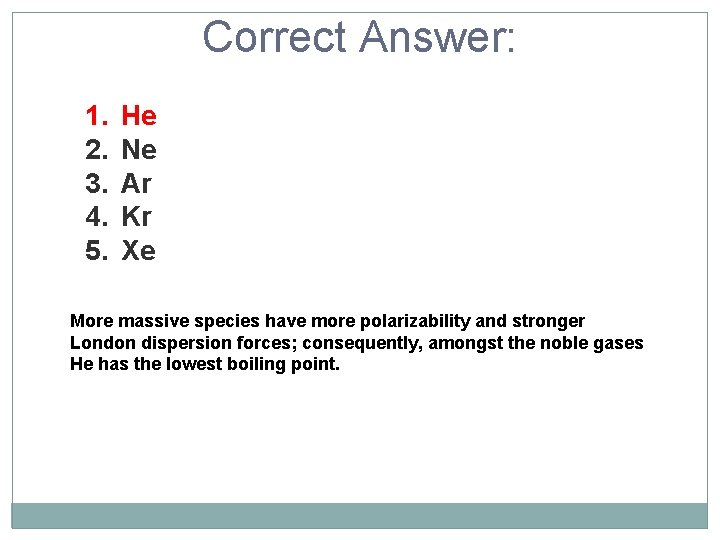Correct Answer: 1. 2. 3. 4. 5. He Ne Ar Kr Xe More massive species have more polarizability and stronger London dispersion forces; consequently, amongst the noble gases He has the lowest boiling point.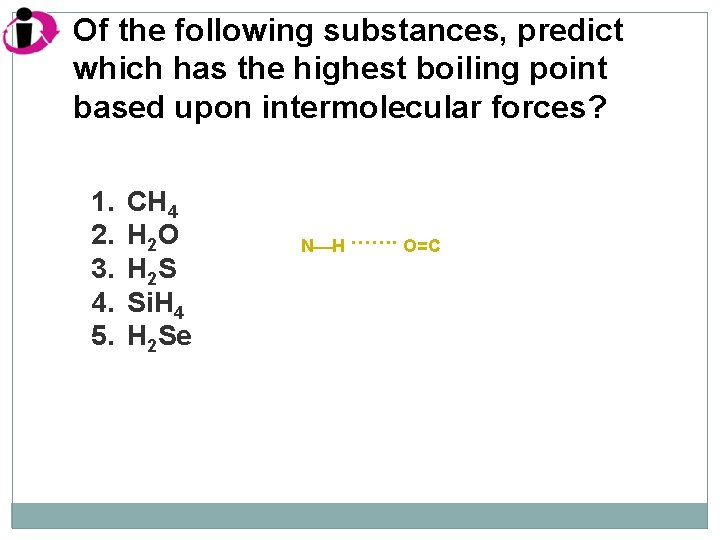Of the following substances, predict which has the highest boiling point based upon intermolecular forces? 1. 2. 3. 4. 5. CH 4 H 2 O H 2 S Si. H 4 H 2 Se N H ……. O=C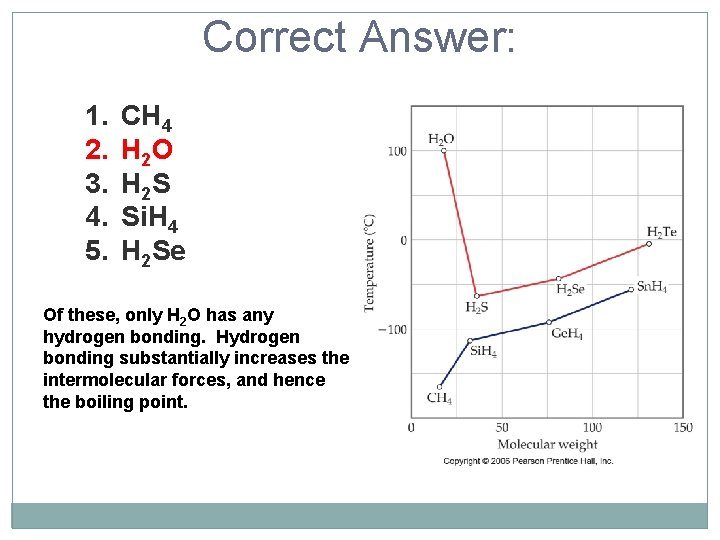Correct Answer: 1. 2. 3. 4. 5. CH 4 H 2 O H 2 S Si. H 4 H 2 Se Of these, only H 2 O has any hydrogen bonding. Hydrogen bonding substantially increases the intermolecular forces, and hence the boiling point.Which one of the following phase changes is an exothermic process? 1. 2. 3. 4. Sublimation Vaporization Condensation Melting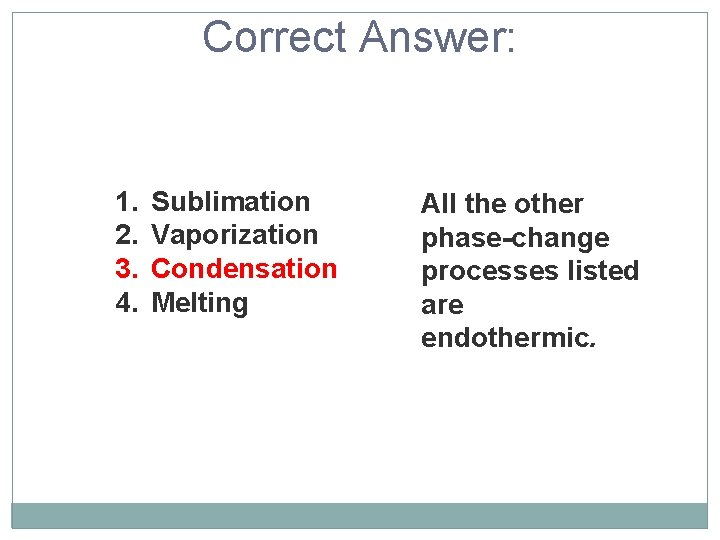Correct Answer: 1. 2. 3. 4. Sublimation Vaporization Condensation Melting All the other phase-change processes listed are endothermic.In the unlabeled phase diagram below, the line segment from A to B separates which two phases? 1. Gas-liquid 2. Liquid-solid 3. Solid-gas Pressure D B A C TemperatureCorrect Answer: 1. Gas-liquid 2. Liquid-solid 3. Solid-gas Pressure D B A C TemperatureQuartz is an example of which type of solid: crystalline or amorphous? 1. Crystalline 2. Amorphous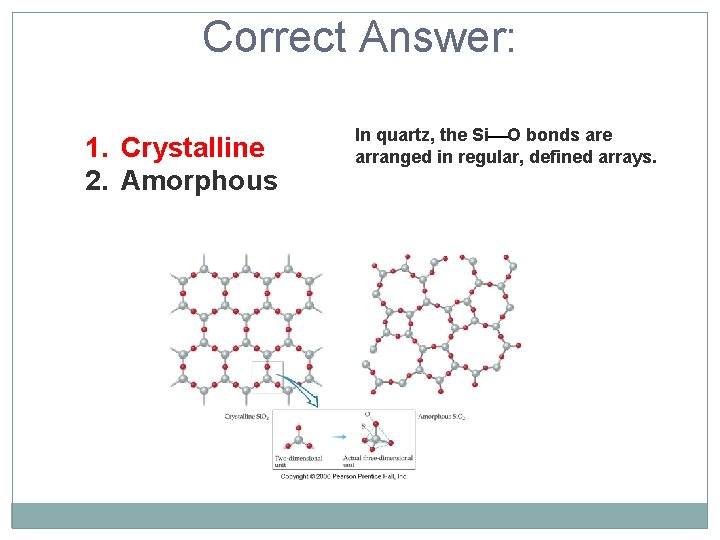Correct Answer: 1. Crystalline 2. Amorphous In quartz, the Si O bonds are arranged in regular, defined arrays.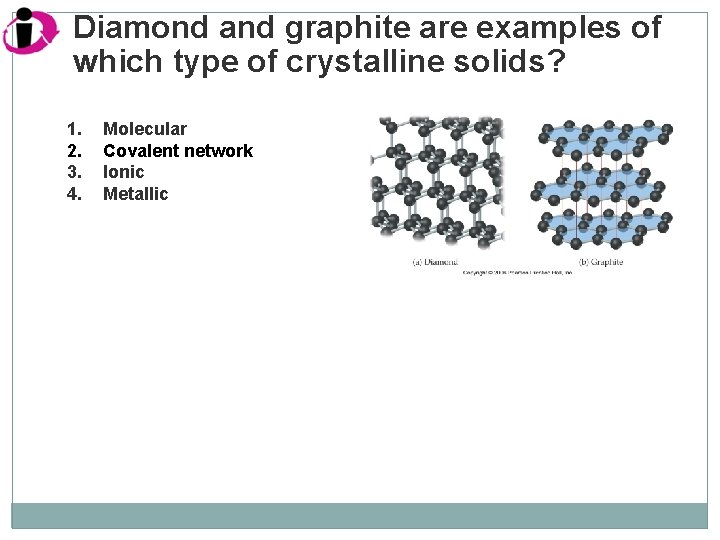Diamond and graphite are examples of which type of crystalline solids? 1. 2. 3. 4. Molecular Covalent network Ionic MetallicCorrect Answer: 1. 2. 3. 4. Molecular Covalent network Ionic Metallic Diamond and graphite are both forms of carbon, and consist of a network of covalent bonds (hence covalent network).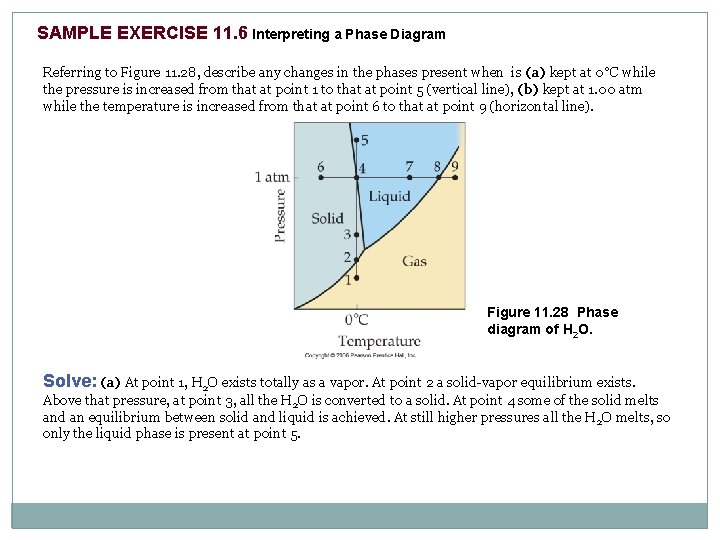SAMPLE EXERCISE 11. 6 Interpreting a Phase Diagram Referring to Figure 11. 28, describe any changes in the phases present when is (a) kept at 0°C while the pressure is increased from that at point 1 to that at point 5 (vertical line), (b) kept at 1. 00 atm while the temperature is increased from that at point 6 to that at point 9 (horizontal line). Figure 11. 28 Phase diagram of H 2 O. Solve: (a) At point 1, H 2 O exists totally as a vapor. At point 2 a solid-vapor equilibrium exists. Above that pressure, at point 3, all the H 2 O is converted to a solid. At point 4 some of the solid melts and an equilibrium between solid and liquid is achieved. At still higher pressures all the H 2 O melts, so only the liquid phase is present at point 5.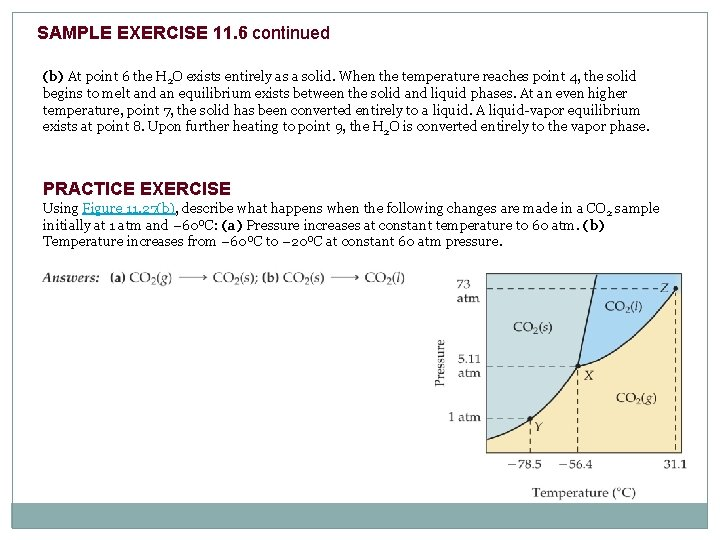SAMPLE EXERCISE 11. 6 continued (b) At point 6 the H 2 O exists entirely as a solid. When the temperature reaches point 4, the solid begins to melt and an equilibrium exists between the solid and liquid phases. At an even higher temperature, point 7, the solid has been converted entirely to a liquid. A liquid-vapor equilibrium exists at point 8. Upon further heating to point 9, the H 2 O is converted entirely to the vapor phase. PRACTICE EXERCISE Using Figure 11. 27(b), describe what happens when the following changes are made in a CO 2 sample initially at 1 atm and – 60ºC: (a) Pressure increases at constant temperature to 60 atm. (b) Temperature increases from – 60ºC to – 20ºC at constant 60 atm pressure.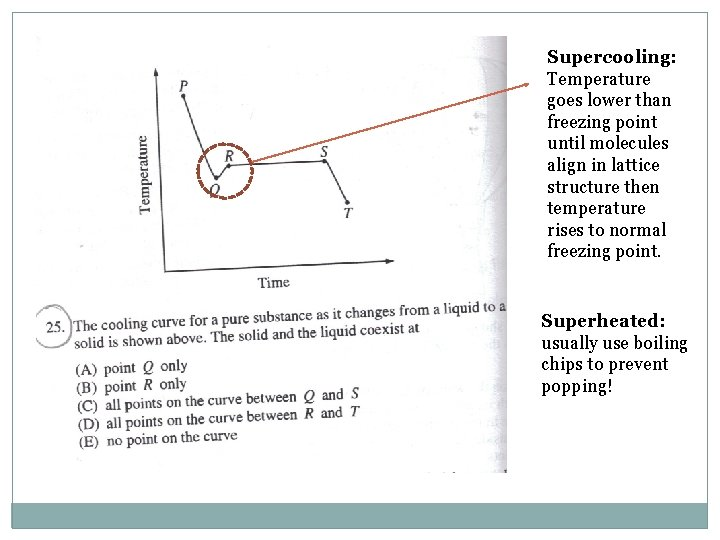Supercooling: Temperature goes lower than freezing point until molecules align in lattice structure then temperature rises to normal freezing point. Superheated: usually use boiling chips to prevent popping!